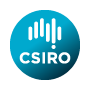# Software from Alan J. Miller

 Index Applied statistics algorithms Quadruple precision code (F) Quadruple precision Random Toms

## Quadruple Precision Code

• quad.f The main module for quadruple precision.
N.B. This version includes functions for complex arithmetic. I have only tested it with the Windows version of F. I am told that it also works with Linux on PCs. On machines which do not use the Intel (or similar) CPU with an 80-bit FPU, try deleting that `11' from the expression for the real (DP), parameter :: const
The IEEE double precision format has a 53-bit mantissa, compared with 64 bits for the Intel FPU, hence that 11. Problems with other computers are most likely to occur with the exponential and trig. functions, so make sure that you test these.
• t_quad.f A simple test program. Various test quantities, e.g. (a^2 - b^2) = (a-b)*(a+b) are calculated in two different ways and the results compared. All of the differences should be of the order of 10^(-30) or zero.
• t_logexp.f and t_cst.f Simple tests of the logarithmic and trigonometric functions.
• q_erf.f The error function and its complement. The file includes a test program which differences the calculated values and compares it with the derivative.
• q_lngam.f The log-gamma function, including a test program.
• q_pzeros.f A module for calculating the zeroes of polynomials. There is also a demo program q_poly20.f.

## Other code for F

• krout.f A module for solving linear equations and inverting matrices.
• lsq.f A module for multiple linear regression using least squares, based upon Applied Statistics algorithm AS 274. There is a demo program which requires a data set